# Texas Go Math Grade 8 Lesson 6.1 Answer Key Identifying and Representing Functions

Refer to our Texas Go Math Grade 8 Answer Key Pdf to score good marks in the exams. Test yourself by practicing the problems from Texas Go Math Grade 8 Lesson Texas Go Math Grade 8 Lesson 6.1 Answer Key Identifying and Representing Functions.

## Texas Go Math Grade 8 Lesson 6.1 Answer Key Identifying and Representing Functions

Essential Question
How can you identify and represent functions?

Explore Activity
Understanding Relationships
Carlos needs to buy some new pencils from the school supply store at his school. Carlos asks his classmates if they know how much pencils cost. Angela says she bought 2 pencils for $0.50. Paige bought 3 pencils for$0.75, and Spencer bought 4 pencils for $1.00. Carlos thinks about the rule for the price of a pencil as a machine. When he puts the number of pencils he wants to buy into the machine, the machine applies a rule and tells him the total cost of that number of pencils.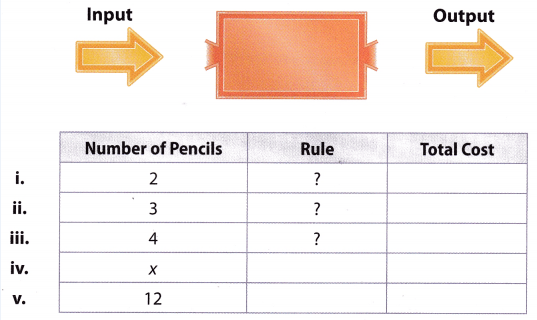A. Use the prices in the problem to fill in total cost in rows i – iii of the table. B. Describe any patterns you see. Use your pattern to determine the cost of 1 pencil. C. Use the pattern you identified to write the rule applied by the machine. Write the rule as an algebraic expression and fill in rule column row iv of the table. D. Carlos wants to buy 12 pencils. Use your rule to fill in row v of the table to show how much Carlos will pay for 12 pencils. Reflect Question 1. How did you decide what operation to use in your rule? Answer: The rule would be to be multiply the number of pencils to the unit rate to find the total cost. Question 2. What If? Carlos decides to buy erasers in a package. There are 6 pencil-top erasers in 2 packages of erasers. Answer: To write the rule as an algebraic expression, we will denote number of pencil-top erasers with variable y and x will represent the package of erasers. a. Write a rule in words for the number of packages Carlos needs to buy to get x erasers. Then write the rule as an algebraic expression. Answer: Knowing that Carlos got six pencil-top erasers in two packages, we can conclude that one package results in three erasers, so we can write it down as: x = $$\frac{y}{3}$$ Therefore, we can claim that for every bought package, Carlos gets three pencil-top erasers. b. How many packages does Carlos need to buy to get 18 erasers? Answer: To calculate how many packages Carlos has to buy to get 18 erasers, we will use given formula as following: x = $$\frac{18}{3}[/latex x = 6 Reflect Question 3. Is it possible for a function to have more than one input value but only one output value? Provide an illustration to support your answer.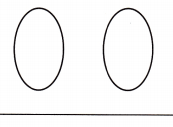Answer: We know that a function assigns exactly one output to each input. Therefore, it is possible for a function to have more than one input value but only one output value. Below, you can find an illustration of this case.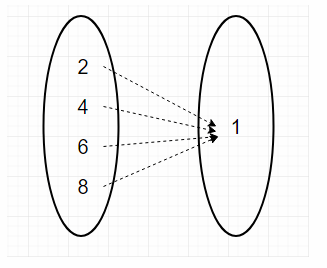Your Turn Determine whether each relationship is a function. Explain. Question 4.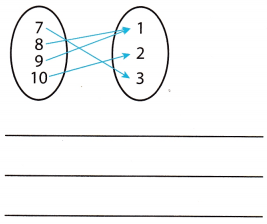Answer: Function A function assigns exactly one output to each input. Question 5.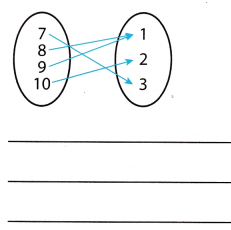Answer: Not function A function assigns exactly one output to each input. Reflect Question 6. What is always true about the numbers in the first column of a table that represents a function? Why must this be true? Answer: To determine the answer here, we will review the definition of a function. In the first column of a table that represents a function there should be no repetition of values. By the definition, table of values represents a function only if each input value has one output value. Therefore, two same values in one column would mean that the table does not represent a function. Your Turn Determine whether each relationship is a function. Explain. Question 7.Answer: Function There is no repetition in the first column. Each input value is paired with only one output value. Question 8.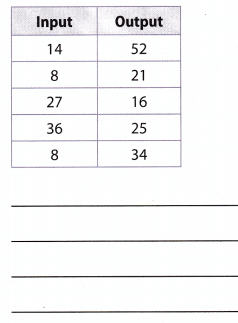Answer: Not a function There is a repetition in the first column which is 8. Hence, 8 has two output 21 and 34. Question 9. Many real-world relationships are functions. For example, the amount of money made at a car wash is a function of the number of cars washed. Give another example of a real-world function. Answer: Profit at the coffee shop can be viewed as a function, considering the fact that it depends on the other variable which is the amount of sold products. Therefore, we can say that the profit is a function of the number of sold products Your Turn Question 10. The graph shows the relationship between the heights and weights of the members of Team Members of a basketball team. Is the relationship represented by the graph a function? Explain.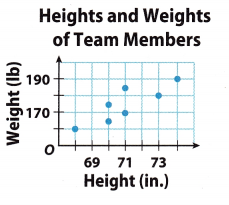Answer: Not a function Input values are paired with more than one output values (70, 165) and (70, 174). Texas Go Math Grade 8 Lesson 6.1 Guided Practice Answer Key Complete each table. In the row with x as the input, write a rule as an algebraic expression for the output. Then complete the last row of the table using the rule. (Explore Activity) Question 1.Answer: Unit cost of ticket = [latex]\frac{40}{2}$$ = 20 Total Cost = 20x where$x$is the number of tickets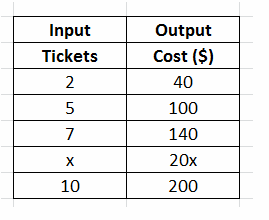Question 2.Answer: Number of pages per minute = $$\frac{1}{2}$$ = 0.5 Total Cost = 0.5x where$x$is the number of minutes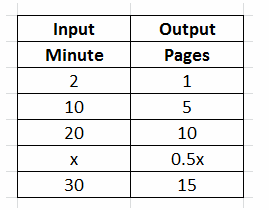Question 3.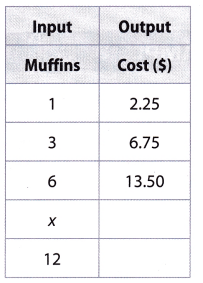Answer: Unit cost of Muffins = $$\frac{2.25}{1}$$ = 2.25 Total Cost = 2.25x where$x$is the number of muffins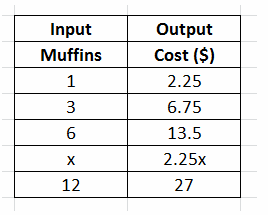Determine whether each relationship is a function. (Examples 1 and 2) Question 4.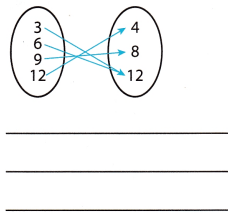Answer: Function Each input is assigned to exactly one output Question 5.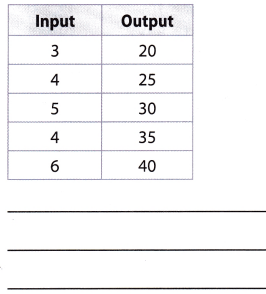Answer: To determine the answer here, we will review the definition of a function. If a certain table of values represents a function, there should be no repetition of values in the first column because in a function each input value is paired with one output value. Therefore, given relationship does not represent a function, because value 4 repeats two times in the first column and results in two output values. Question 6. The graph shows the relationship between the weights of 5 packages and the shipping charge for each package. Is the relationship represented by the graph a function? Explain.Answer: Explanation A: Because inputs are paired with output value and there is no repeated value, the relationship is a function. Explanation B: To determine the answer, we will write values from the graph in one table. First column represents the weight and the second one represents the shipping cost. Table is shown below.Considering the fact that there is no repetition of input values and every input value is paired with one output value, we can claim that given relationship is a function. Essential Question Check-In Question 7. What are four different ways of representing functions? How can you tell if a relationship is a function? Answer: A function is defined as the relationship in which the input value is paired with exactly one output value. In representing functions, we have four different ways. These are using Mapping Diagrams, Tables, Graphs, and Equations. A mapping diagram represents a function if each input value is paired with only one output value. Relationships of functions between input values and output values can also be represented using tables. The values in the first column are the input values. The values in the second column are the output values. Graphs can be used to displays relationships between two sets of numbers. Each point on a graph represents an ordered pair A function can be represented by an equation with two variables. One variable is the domain and the other is the range. By the description, the four ways of representing a function are through Mapping Diagrams, Tables, Graphs, and Equations Also, we can say that a relationship is a function if the input value is paired with exactly one output value. Texas Go Math Grade 8 Lesson 6.1 Independent Practice Answer Key Determine whether each relationship represented by the ordered pairs is a function. Explain. Question 8. (2, 2), (3, 1), (5, 7), (8, 0), (9, 1) Answer: A function is defined as a relation such that the input value is paired with exactly one output value. To determine if the given ordered pairs (2, 2), (3, 1), (5, 7), (8, 0), (9, 1) is a function, we can write it in table form, wherein x-coordinate is represented as input and y-coordinate is represented as output. |input (x) | Output(y)| |——|——| |2 |2| |3|1| |5 | 7| |8| 0| | 0|1| As we can notice in the table, one output value is paired with two input values these are (3, 1) and (0, 1), however each input value is paired only with one output value, so still the relationship is a function. A function; Each input value is paired only with one output value. Question 9. (0, 4), (5, 1), (2, 8), (6, 3), (5, 9) Answer: A function is defined as a relation such that the input value is paired with exactly one output value. To determine if the given ordered pairs (0,1), (5, 1), (2,8), (6,3), (5,9) is a function, we can write it in table form, wherein x-coordinate is represented as input and y-coordinate is represented as output |Input (x) | Output (y)l |–|–| |0|4| |5|1| |2 | 8| |6| 3| |5| 9| As we can notice in the table, one input value “5” is paired with two output values “1 and 9”, (5, 1) and (5, 9), so the relationship is not a function. Not a function; The input value 5 is paired with more than one output value 1 and 9. Question 10. Draw Conclusions Joaquin receives$0.40 per pound for 1 to 99 pounds of aluminum cans he recycles. He receives $0.50 per pound if he recycles more than 100 pounds. Is the amount of money Joaquin receives a function of the weight of the cans he recycles? Explain your reasoning. Answer: Yes The amount of money increases with the weight of the cans. No weight will result in the same amount of money earned. Question 11. A biologist tracked the growth of a strain of bacteria, as shown in the graph.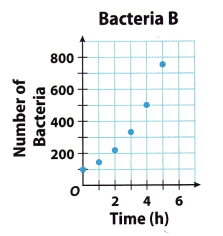a. Explain why the relationship represented by the graph is a function. Answer: Relationship is a function as each input has been assign exactly one ouput. There is only one number of bacteria for each number of hours. b. What It? Suppose there was the same number of bacteria for two consecutive hours. Would the graph still represent a function? Explain. Answer: Yes. If the number of bacteria for two consecutive hours is the same, one input will stilt be paired with one output hence the relationship is still a function. Question 12. Multiple Representations Give an example of a function in everyday life, and represent it as a graph, a table, and a set of ordered pairs. Describe how you know it is a function.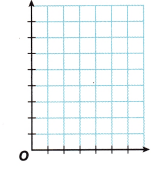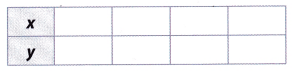Answer: The cost of a bouquet of flowers and the number of flowers in the bouquet is a function. Unit cost of flowers =$0.85 and x is the number of flowers. Hence. C = 0.85x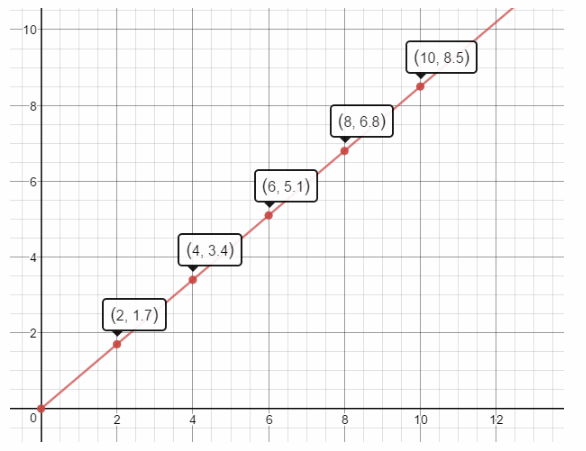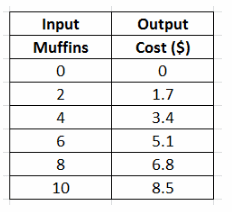Ordered Pairs
(2, 1.7), (4, 3.4), (6, 5.1), (8, 6.8), (10, 8.5)
Each value of input is paired with exactly one output.

The graph shows the relationship between the weights of six wedges of cheese and the price of each wedge.

Question 13.
Is the relationship represented by the graph a function? Justify your reasoning. Use the words ‘input” and output” in your explanation, and connect them to the context represented by the graph.Yes, the relationship represented by the graph is a function. Each input (weight) in the graph is paired with exactly one output (price).

Yes, the relationship represented by the graph is a function.

Question 14.
Analyze Relationships Suppose the weights and prices of additional wedges of cheese were plotted on the graph. Is that likely to change your answer to question 13? Explain your reasoning.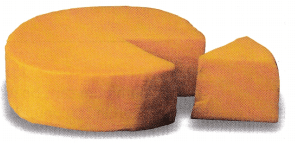Explanation A:
No, since the weight of the cheese is directly proportional to the cost of wedges of cheese.

Explanation B:
If weights and prices of additional wedges of cheese were plotted on the graph, it would still result in a function

Relationship would still result in a function because the price of wedges and weight are proportional values, meaning that for every input (weight) there would be only one output (price).

Texas Go Math Grade 8 Lesson 6.1 H.O.T Focus On Higher Order Thinking Answer Key

Question 15.
Justify Reasoning A mapping diagram represents a relationship that contains three different input values and four different output values. Is the relationship a function? Explain your reasoning.